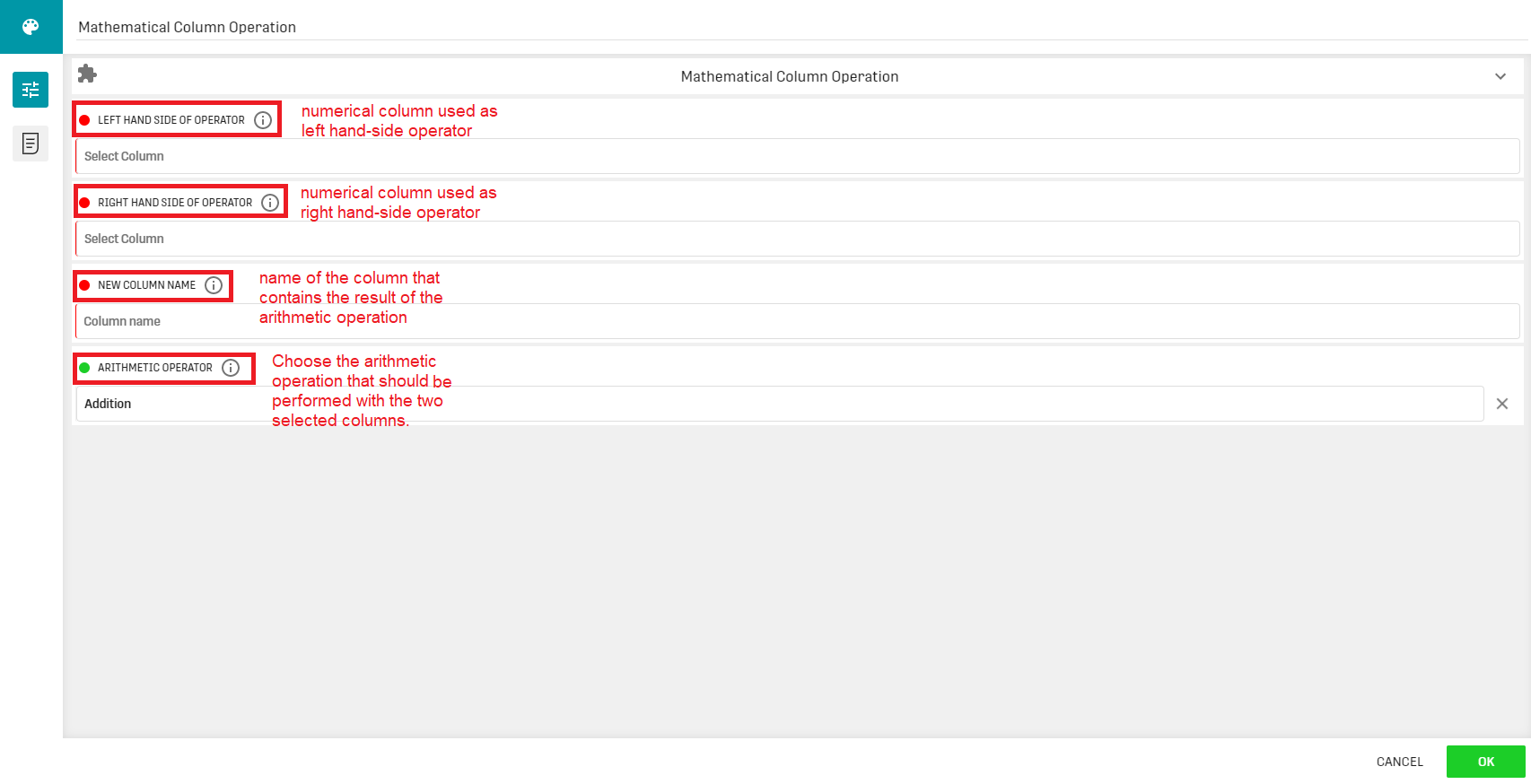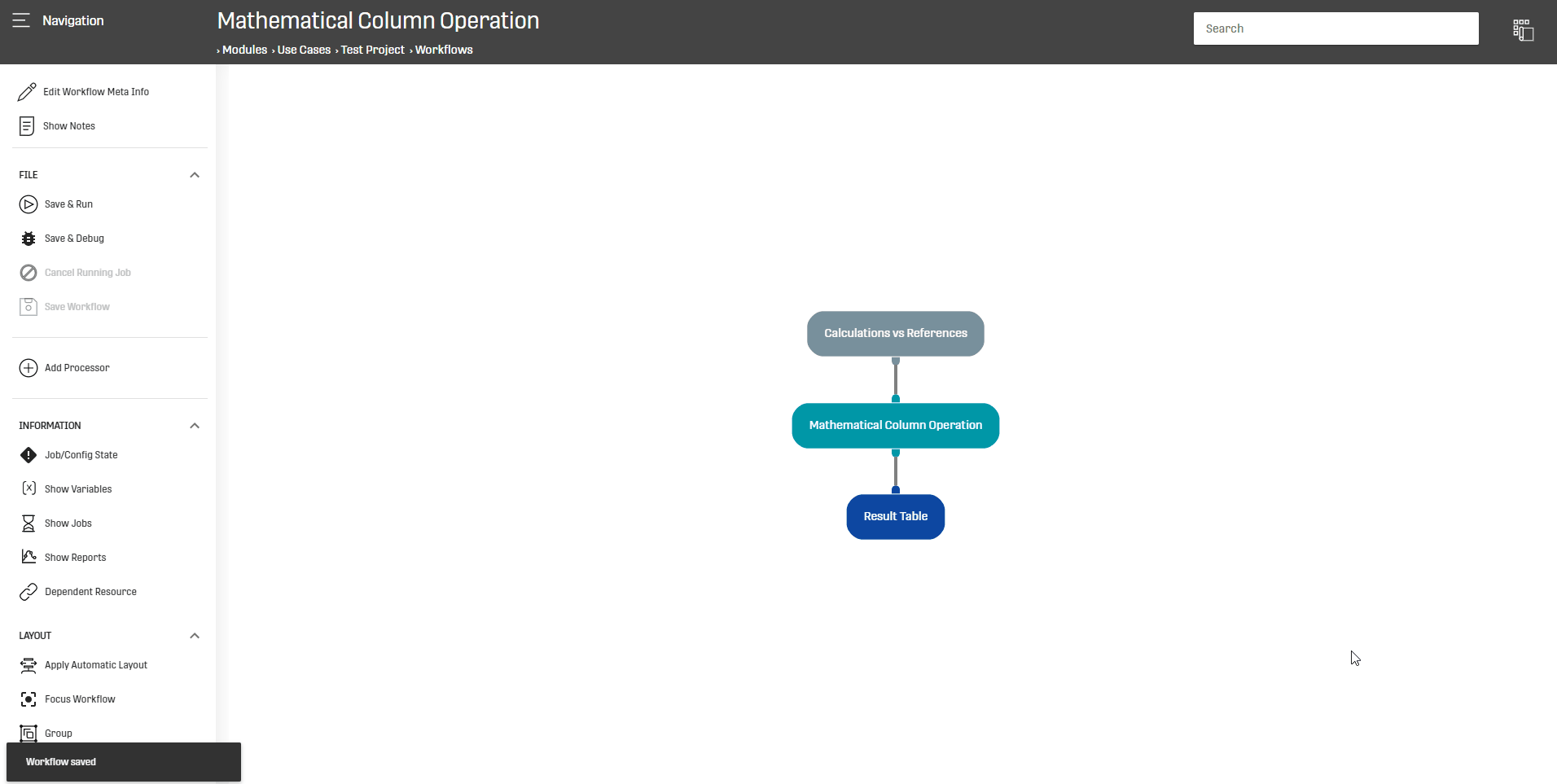# Mathematical Column Operation Processor

Modified on Tue, 30 Nov 2021 at 02:27 PM

# Overview

This processor is used to execute an elementary arithmetic operation between two selected columns and saves the result in a new column.

# Configuration

This processor operates on any given dataset containing at least one numerical column.

The configuration menu of the processor looks as follows:The possible arithmetic operations are: Addition, Division, Multiplication and Subtraction.

# Output

The output of this processor is the initial dataset along with the new column containing the computed entries.

BE AWARE THAT: The column containing the computed entries can have the same name as a column of a column from the input dataset (so the column values will be overwritten).

# Example

In this scenario, the Mathematical Column Operation Processor will be used to compute the difference between the entries of two columns from an input dataset:# Related Articles

Extended Mathematical Operation

Mathematical Timestamp Operation Processor

Mathematical Operation MC Processor

Query Processor